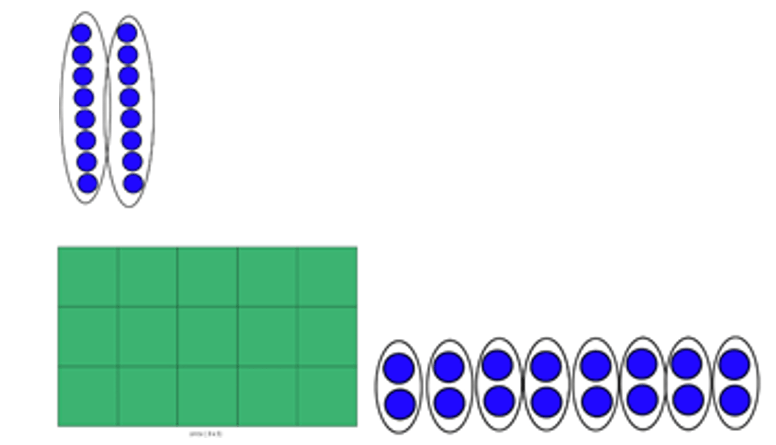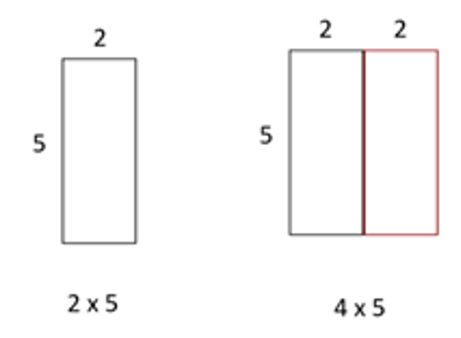## Visualizing Middle Years

Visualizing helps students investigate the difference between two groups of eight ( 2 x 8) and eight groups of two ( 8 x 2). An array (3 x 5) connects students to an area model that can be used throughout K-12.N4.3, N4.4:

Outcomes N4.3 and N4.4 are both focused on student understanding of multiplication. The outcome N4.3 asks students to explain multiplication strategies. Students can and should explain multiplication strategies using visual models. Different models are used to show different thinking and illustrate different strategies.

Example:Students use an area model as a visual to show that you double the area in order to find 4 x 5. Using an area model also connects students to the area outcome that is also in grade 4.

Students might use a number line visual to indicate that they used a skip counting strategy.

Different models are used to show different thinking and illustrate different strategies. Students make the choice of what visual model to use. This choice illustrates for the teacher how students are thinking about multiplication strategies and therefore informs future instructional choices.The purpose of learning multiple strategies is not for students to be able to list the different strategies, but rather to look at any question they are given and make a decision on a strategy that is most efficient. As students become more flexible and fluent with strategies, they build number sense.

Answering questions where the teacher has dictated the strategy that students must use or asking students to explain an already provided strategy does little to build or assess student number sense.

For example:

I know that 10 x 3 = 30 and 30 – 3 = 27. Therefore, 9 x 3 = 27.

Explain the strategy that was used to solve the question 9 x 3 = 27.

In the above question, most of the thinking that would build number sense is already done for the student. There are no visuals for the student to connect to and the answer the student provides only informs the teacher if the student can recognize a provided strategy.